# MCAT Physical : Centripetal Force and Acceleration

## Example Questions

### Example Question #1 : Centripetal Force And Acceleration

An insect sits at the edge of slowly turning wheel.  The wheel is accelerated gradually until the insect can no longer hold on.  The insect’s path of travel will be:

1. The insect will move away from the wheel in a straight line perpendicular to the wheel edge.
2. The insect will move away from the wheel in a straight line tangent to the wheel edge.
3. The insect will move away from the wheel edge in a curvilinear path.
4. The path is not predictable by Newtonian physics.
5. None of these is true.
Possible Answers:

4

5

3

1

2

Correct answer:

2

Explanation:

Choice 2 is correct; this is how a classical slingshot operates. A body in motion tends  to remain in motion unless a force disturbs that motion. At any point in time, the insect is moving in a straight line along a line tangent to the circumference of the wheel. When the little creature leaves the wheel, there is no longer any force acting on it, so it will move in a straight line, not a curvilinear path. Newtonian kinetics of course includes these concepts, because they relate closely to the motion of celestial bodies.

### Example Question #2 : Centripetal Force And Acceleration

For an object traveling in a circle at a constant velocity of 100m/s, which of the following is true?

Possible Answers:

The radius of the circle is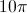No forces are acting on the object

Only gravitational force is acting on the object

The object is undergoing constant acceleration

Correct answer:

The object is undergoing constant acceleration

Explanation:

Since the object is traveling in a circle, it is constantly changing direction. This means that the direction of the velocity is also changing, even if the magnitude is not. Because the magnitude of the velocity does not change, we can assume that acceleration is constant.

### Example Question #3 : Centripetal Force And Acceleration

A ball with a mass of 0.95kg is attached to a string with a length of 75cm and moves in a vertical circle at a constant speed of 25m/s. What is the maximum tension in the string?

Possible Answers:

800N

30N

790N

40N

Correct answer:

800N

Explanation:

Maximum tension occurs when the ball is at its bottommost point, as the string experiences both the downward force of gravity and centripetal force.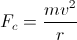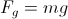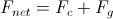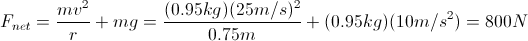### Example Question #4 : Centripetal Force And Acceleration

Two identical cars, A and B, are traveling on identical surfaces. Car A drives at a constant velocity in a perfectly circular path. Car B drives at a constant velocity along a long, straight path. Which best describes the accelerations,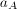and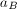, of the two cars?

Possible Answers: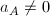and is radially outwards from the center of its path;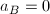and is in the same direction as car A's velocity;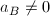and is in the same direction as car B's velocity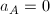and is in the same direction as car B's velocityand is radially inwards towards the center of its path;Correct answer:and is radially inwards towards the center of its path;Explanation:

An object moving in constant circular motion must have centripetal acceleration, directed towards the center of its path, in order to maintain its circular motion. So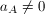and is towards the center of car A's path.

An object moving linearly at constant velocity has 0 acceleration, so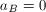.

In general, we can think of acceleration as a change in either the magnitude or direction of an object's velocity. Neither of these cars has a change in the magnitude of its velocity, but car A does experience a change in the direction of its velocity, while car B does not.

### Example Question #5 : Centripetal Force And Acceleration

What is the approximate centripetal acceleration of a point on the rim of a bicycle wheel with a diameter of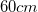if the wheel is rotating at eight revolutions per second?

Possible Answers: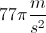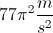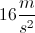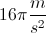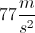Correct answer:Explanation:

Centripetal acceleration is given by the equation: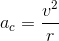We are given the angular velocity and the diameter. We can easily solve for the radius from the diameter, and use the angular velocity to find the linear velocity.

Convert the angular velocity to the proper units by changing revolutions to radians.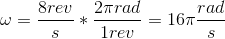Now we can convert the angular velocity to the linear velocity using the radius.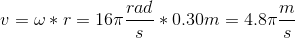Use the radius and the linear velocity to calculate the centripetal acceleration from the original equation.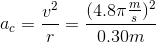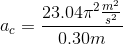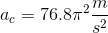### Example Question #6 : Centripetal Force And Acceleration

What is the centripetal force on a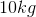ball being swung in a vertical loop with a velocity of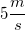on a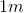string?

Possible Answers: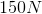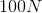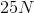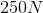Correct answer:Explanation:

Centripetal force is given by the equation: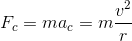The length of the string represents the radius of the circle being formed. Use the given string length as the radius, along with the mass of the ball and the velocity, to calculate the centripetal force.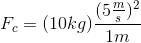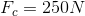### Example Question #7 : Centripetal Force And Acceleration

A 2kg mass is suspended on a rope that wraps around a frictionless pulley. The pulley is attached to the ceiling and has a mass of 0.01kg and a radius of 0.25m. The other end of the rope is attached to a massless suspended platform, upon which 0.5kg weights may be placed. While the system is initially at equilibrium, the rope is later cut above the weight, and the platform subsequently raised by pulling on the rope.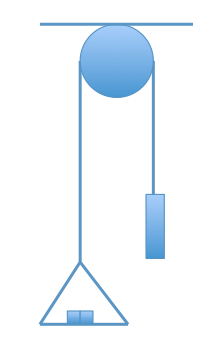What is the linear velocity of the pulley the instant the rope to the 2kg mass is cut?

Possible Answers: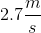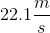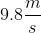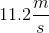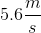Correct answer:Explanation:

Note that we are asked the linear velocity of the pulley, not the angular velocity. With Newton’s second law, we know that F = ma. Additionally, in rotational motion, we know that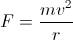. The force (F) the instant the rope is cut is due to the platform with the 4 weights. Knowing the mass and radius of the pulley, we can solve for the linear velocity: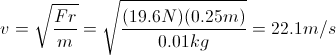### Example Question #8 : Centripetal Force And Acceleration

A man of mass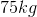is riding a roller coaster, which has a total mass of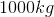. As the ride enters a circular loop, it is traveling at a rate of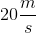. The loop has a radius of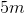. If the average frictional force on the coaster is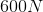, how many g's of force does the man experience at the top of the loop?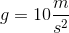Possible Answers: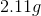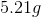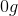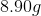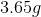Correct answer:Explanation:

To calculate g's, we need to know the centripetal force the rider is experiencing. To calculate centripetal force, we need to know how fast the ride is traveling at the top of the loop. To do this, we will use the equation for conservation of energy: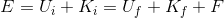If we set the height of the top of the loop to 10m, we can say that there is no initial potential energy. Since we are solving for final velocity, let's rearrange this for final kinetic energy: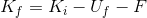Plugging in the expressions for each of these terms, we get: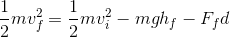Rearranging for final velocity: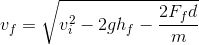We have all of the values except for how far the coaster travels. It travels from the bottom to the top of the loop, which is half the circumference of the circle: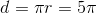Now we can plug in our values and solve: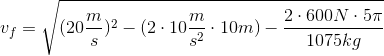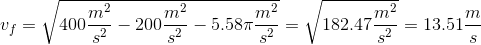Now that we know how fast the ride is traveling at the top of the loop, we can calculate the centripetal force on the man: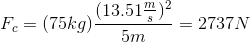Now that we know the force in Newtons, we can convert this value to g's.

For this man: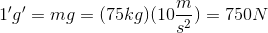Therefore,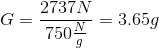### All MCAT Physical Resources